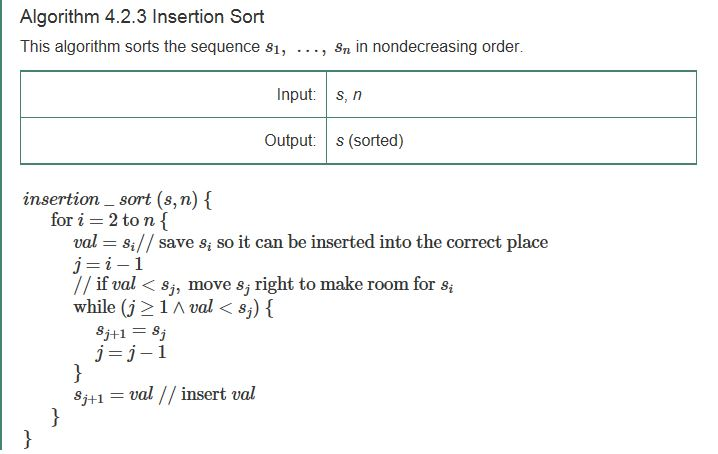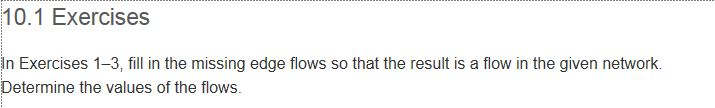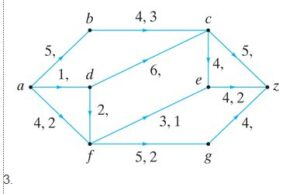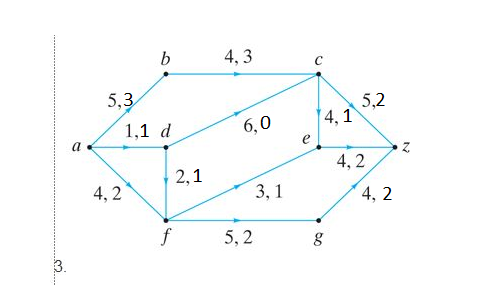# Discrete Mathematics Solved Assignment Solution Sample

QUESTION

• Data Storage, Retrieval, Sorting Algorithms, Time Complexity

In computer systems, the storage, retrieval, and communication of data occurs frequently and therefore must be optimized and fast. In some instances, it is best to store a list of numbers in some order (for example, in an increasing or decreasing order), to make the retrieval of some of the data very fast (for example, the maximum value).

In section 4.2 of your textbook, you read about the insertion sort algorithm (see Algorithm 4.2.3, page 180). This algorithm will sort a list of n numbers in non-decreasing order.In order to fully understand this question, answer the following questions:

1. The name of the faster sorting algorithm is the tournament sort. How is this faster sorting algorithm achieved?
2. Given a list of n numbers, what is the worst case times for the insertion sort algorithm and the tournament sort algorithm?
3. In the insertion sort and the tournament sort algorithms, what discrete structures are used to store the data? Explain.
4. How does the use of the tree structure provide the framework for a tournament sort?
5. What is a divide-and-conquer algorithm? Is this a divide-and-conquer algorithm?

Section 10.1, page 510, exercise 3• Data Storage, Retrieval, Sorting Algorithms, Time Complexity

In computer systems, the storage, retrieval, and communication of data occurs frequently and therefore must be optimized and fast. In some instances, it is best to store a list of numbers in some order (for example, in an increasing or decreasing order), to make the retrieval of some of the data very fast (for example, the maximum value).

In section 4.2 of your textbook, you read about the insertion sort algorithm (see Algorithm 4.2.3, page 180). This algorithm will sort a list of n numbers in non-decreasing order.In order to fully understand this question, answer the following questions:

1. The name of the faster sorting algorithm is the tournament sort. How is this faster sorting algorithm achieved?

ANS: Tournament sort is a faster sorting technique because of the application of min heap which uses a binary tree for deciding the winner element and i(tournament sorting ) is being done in log(n) time only and O(n) operations to select next n elements.

1. Given a list of n numbers, what is the worst case times for the insertion sort algorithm and the tournament sort algorithm?

ANS: Worst case time complexity for insertion sort is O(n2) and worst case time complexity for tournament sort is O(n log n).

1. In the insertion sort and the tournament sort algorithms, what discrete structures are used to store the data? Explain.

ANS: In insertion sort algorithm basically array or linked list is used for storing data because insertion sort is defined for array and lists only if we use any other data structure then it will be some other algorithm.

In tournament sort complete binary tree is used as data structure which is also called as winner tree. Binary tree is used for creation of min heap whose root element creates the element for sorted list.

1. How does the use of the tree structure provide the framework for a tournament sort?

ANS: A tournament sort uses a min heap for its sorting procedure and a min heap is a version of a binary tree hence the tree structure provides the framework for a tournament sort.

1. What is a divide-and-conquer algorithm? Is this a divide-and-conquer algorithm?

ANS: Divide-and-conquer algorithm is an algorithmic paradigm which works recursively and solves a problem by dividing a problem into sub-problems and recombines those smaller problems to gain original problem’s solution. It is basically divided into three sub-parts called as divide , conquer and combine.

The insertion sort is not a divide and conquer algorithm.

Section 10.1, page 510, exercise 3